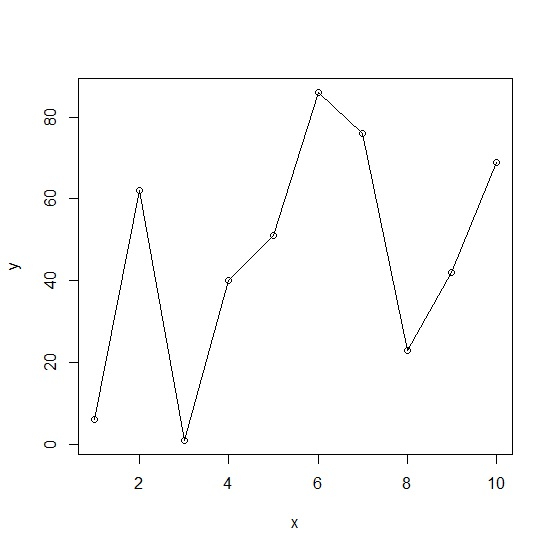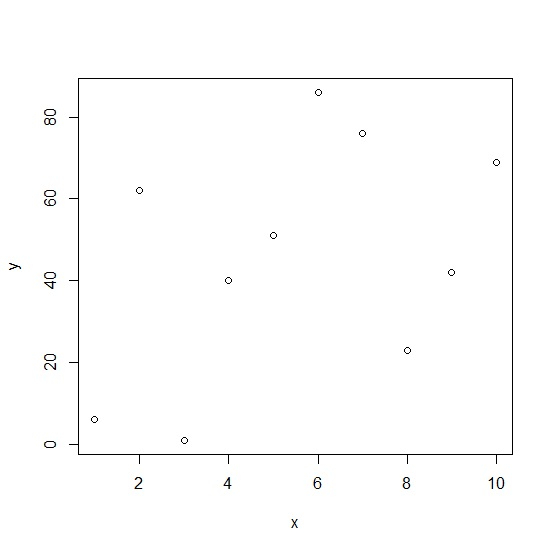# How to delete lines after creating a line chart with points in base R?

To delete lines after creating a line chart with points in base R we would need to create the points chart only, therefore, we can follow the below steps −

• First of all, create two vectors and create the line chart with points.
• Now, again create the line chart but without points

## Create the vectors and line chart with points

Use plot and lines function to create the line chart with points as shown below −

Live Demo

x<-1:10
y<-sample(1:100,10)
plot(x,y)
lines(x,y)

On executing, the above script generates the below output(this output will vary on your system due to randomization) −

### Output## Create the points chart without lines

Use plot function to create the point chart without lines as shown below −

Live Demo

x<-1:10
y<-sample(1:100,10)
plot(x,y)

### Output